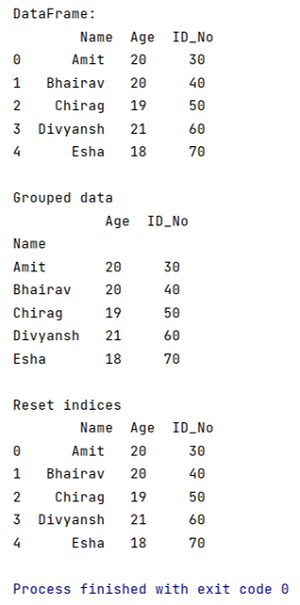# How to flatten a hierarchical index in columns?

Learn how to flatten a hierarchical index in columns? By Pranit Sharma Last updated : September 19, 2023

While creating structured grouped data in pandas, we use the hierarchical indexing method. These hierarchical indexes are useful for complex data queries. Here, we are going to learn how to flatten these hierarchical indices in columns.

## Flatten a hierarchical index in columns

Pandas allows us to achieve this task by using the reset_index() method. This method is used to rearrange or reset the hierarchical indices that occurred due to groupby aggregated functions.

### Syntax

```DataFrame.reset_index(
level=None,
drop=False,
inplace=False,
col_level=0,
col_fill=''
)
```

### Parameter(s)

• It takes a parameter called level, if defined then removes the particular levels from the indices.
• It takes another parameter called drop, which is defined to set the flatten index to the default integer index.
• Also, it takes a parameter called inplace which modifies the original DataFrame without creating a copy.
Note

To work with pandas, we need to import pandas package first, below is the syntax:

```import pandas as pd
```

Let us understand with the help of an example:

## Python program to flatten a hierarchical index in columns

```# Importing pandas package
import pandas as pd

# Creating a Dictionary
dict = {
'Name':['Amit','Bhairav','Chirag','Divyansh','Esha'],
'Age':[20,20,19,21,18],
'ID_No':[30,40,50,60,70]
}

# Creating a DataFrame
df = pd.DataFrame(dict)

# Display DataFrame
print("DataFrame:\n",df,"\n")

# Using groupby on Name based on sum of age
result = df.groupby(by='Name').agg(sum)

# Display Result
print("Grouped data\n",result,"\n")

# Reset index
reset = result.reset_index()

# Display reset
print("Reset indices\n",reset)
```

### Output

The output of the above program is: